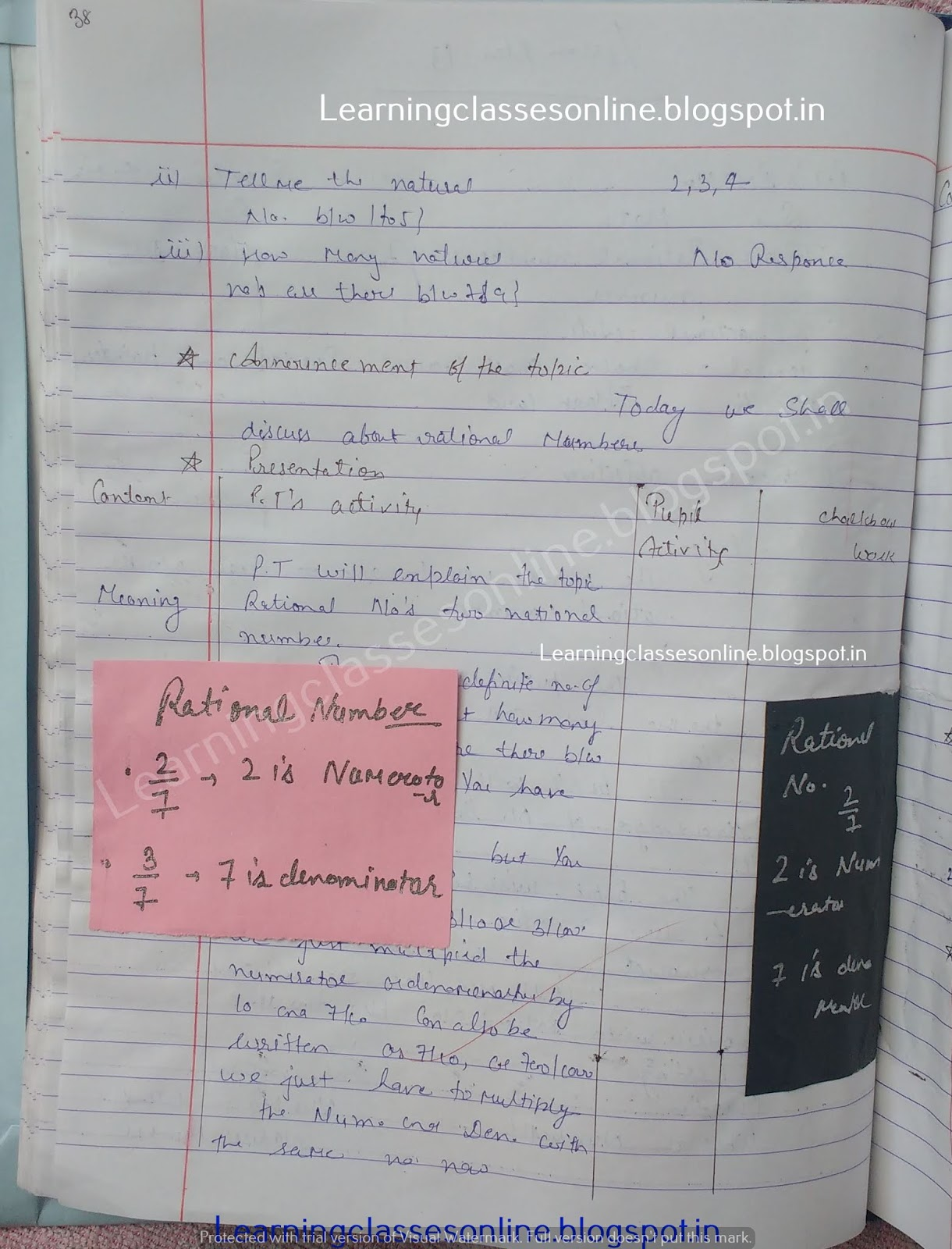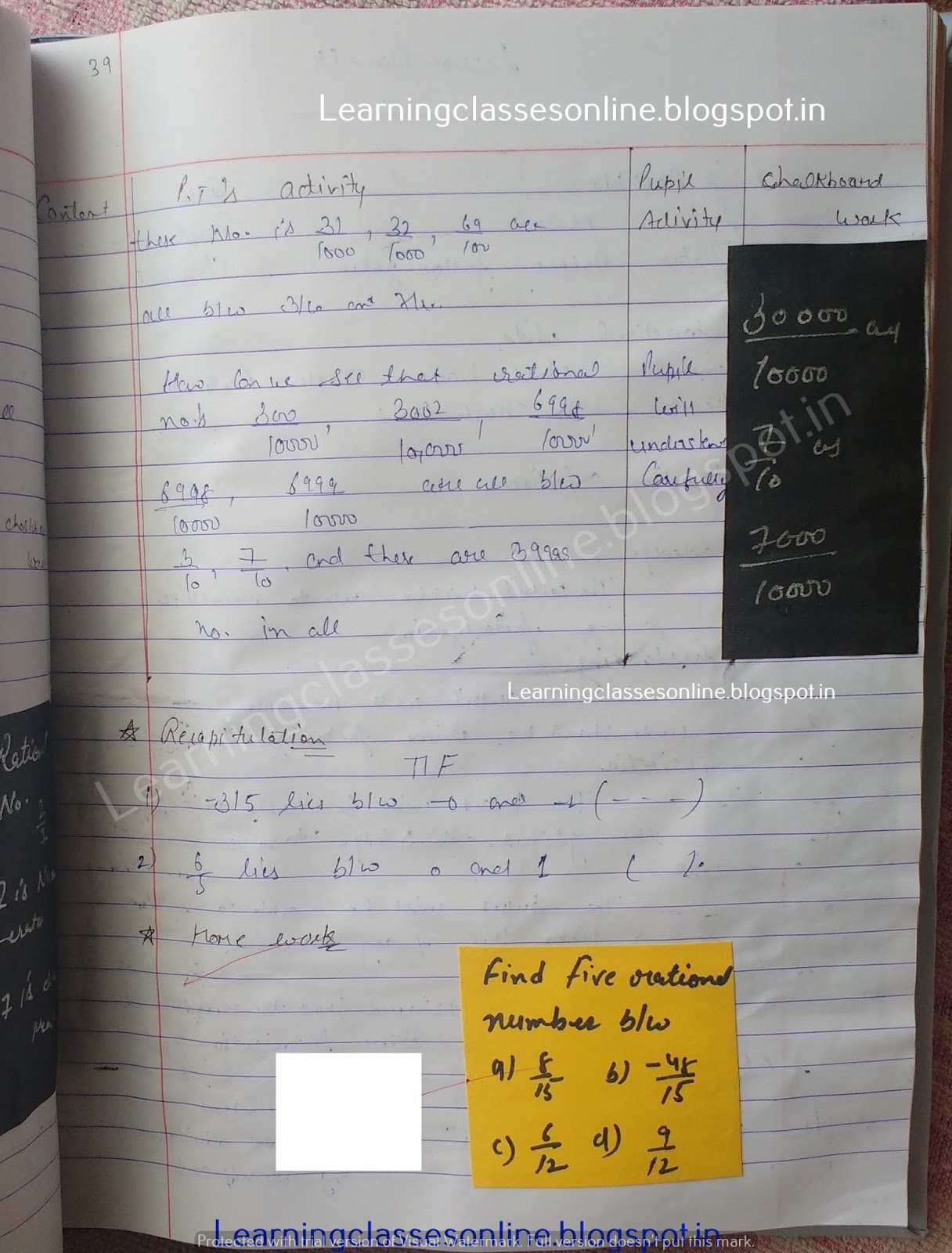Math lesson Plan on Rational numbers between two numbers

# Math lesson Plan on Rational numbers between two numbers

Math lesson Plan on Rational numbers between two for class 6th, 7th, 8th and 9th.

Note: The Mathematics Lesson Plan given below is just an example. You can change the Name, Class, Course, Date, Duration, etc. according to your needs.Further Reference:
www.learningclassesonline.com Mathematics Lesson Plan for B.Ed